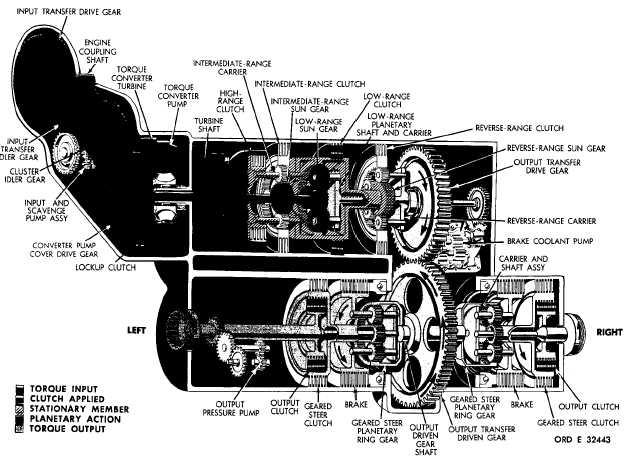Custom SearchP A R   4   8 T O R Q U E     P A T H S C H A P   2,   SEC  V Figure  24.  Second-gear  torque  path,  lockup  operation 48.   TORQUE   PATH   –   SECOND   GEAR, geared   steer   planetary   carriers   and   sun   gears LOCKUP  OPERATION   (fig.   24) together.    When    these    components    are    locked The   torque   path   in   second   gear   is   identical together,   the   ring   gears   drive   them   as   units. to   that   for   first   gear   until   it   leaves   the   geared The   output   speed   is   thus   equal   to   the   speed   of steer   planetary   ring   gears   (refer   to   47a   and   b , the   ring   gears. Refer   to   TABULATED   DATA, a b o v e ) .    I n    s e c o n d    g e a r ,    t h e    r i g h t -    a n d    l e f t- p a r .    7 ,    f o r    g e a r    r a t i o s    a p p l i c a b l e    t o    s e c o nd o u t p u t    c l u t c h e s    a r e    e n g a g e d .    T h i s    l o c k s    t h e g e a r. 5 1